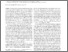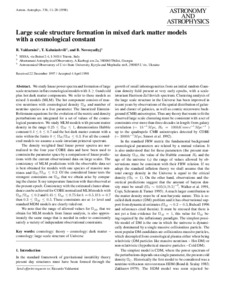# Large scale structure formation in mixed dark matter models with a cosmological constan

Valdarnini, Riccardo and Kahniashvili, Tina and Novosyadlyj, Bohdan (1998) Large scale structure formation in mixed dark matter models with a cosmological constan. Astronomy and Astrophysics, 336. pp. 11-228.Preview
Text
vkn.pdf

Download (547kB) | Preview

## Abstract

We study linear power spectra and formation of large scale structures in flat cosmological models with Lambda >= 0 and cold plus hot dark matter components. We refer to these models as mixed Lambda models (MLM). The hot component consists of massive neutrinos with cosmological density Omega_H and number of neutrino species as a free parameter. The linearized Einstein-Boltzmann equations for the evolution of the metric and density perturbations are integrated for a set of values of the cosmological parameters. We study MLM models with present matter density in the range 0.25 <= Omega_M <= 1, dimensionless Hubble constant 0.4 <= h<= 0.7 and the hot dark matter content with a ratio within the limits 0 <= Omega_H /Omega_M <=0.3. For all the considered models we assume a scale-invariant primeval spectrum. The density weighted final linear power spectra are normalized to the four year COBE data and have been used to constrain the parameter space by a comparison of linear predictions with the current observational data on large scales. The consistency of MLM predictions with the observable data set is best obtained for models with one species of massive neutrinos and Omega_H /Omega_M <=0.2. on the Of the considered linear tests the strongest constraints on Omega_M that we obtain arise by comparing the cluster X-ray temperature function with that observed at the present epoch. Consistency with the estimated cluster abundance can be achieved for COBE normalized MLM models with Omega_H /Omega_M <=0.2 and 0.45 <= Omega_M <= 0.75 for h=0.5. If h=0.7 then 0.3 <= Omega_M <= 0.5. These constraints are at 1sigma level and standard MDM models are clearly ruled out. We note that the range of allowed values for Omega_M , that we obtain for MLM models from linear analysis, is also approximately the same range that is needed in order to consistently satisfy a variety of independent observational constraints.

Item Type: Article Q Science > QB Astronomy Prof. Tina Kahniashvili 27 Jan 2014 08:59 27 Mar 2015 06:52 http://eprints.iliauni.edu.ge/id/eprint/648

### Actions (login required)View Item